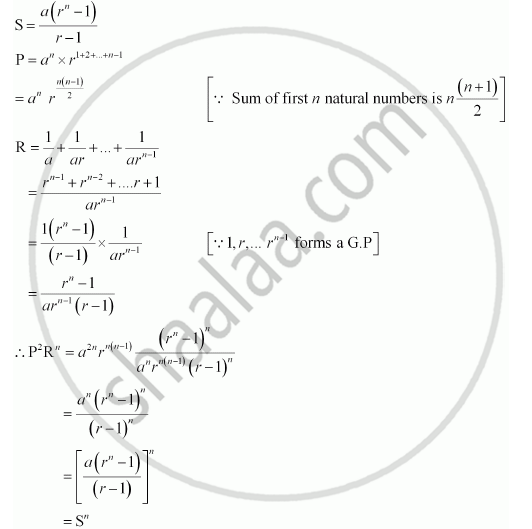Share

# Let S Be the Sum, P the Product and R the Sum of Reciprocals of N Terms in a Geometric Progression . Prove that P2rn = Sn - Mathematics

#### Question

Let S be the sum, P the product and R the sum of reciprocals of n terms in a G.P. Prove that P2Rn = Sn

#### Solution

Let the G.P. be aarar2ar3, … arn – 1

According to the given information,Hence, P2 Rn = Sn

Is there an error in this question or solution?